# Forced Convective Heat Transfer - 2 Notes | Study Heat Transfer - Mechanical Engineering

## Mechanical Engineering: Forced Convective Heat Transfer - 2 Notes | Study Heat Transfer - Mechanical Engineering

The document Forced Convective Heat Transfer - 2 Notes | Study Heat Transfer - Mechanical Engineering is a part of the Mechanical Engineering Course Heat Transfer.
All you need of Mechanical Engineering at this link: Mechanical Engineering

The discussion on heat transfer correlations consists of many dimensionless groups. Therefore, before we discuss the importance of heat transfer coefficients, it is important to understand the physical significance of these dimensionless groups, which are frequently used in forced convection heat transfer. The table 4.1 shows some of the dimensionless numbers used in the forced convection heat transfer.

Table-4.1: Some important dimensionless numbers used in forced heat transfer convection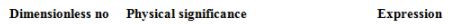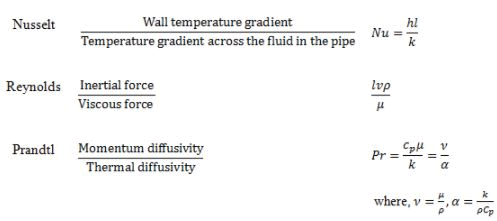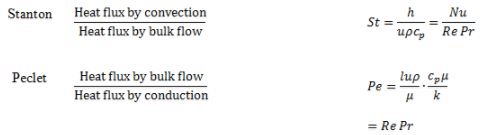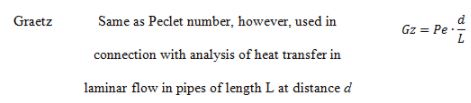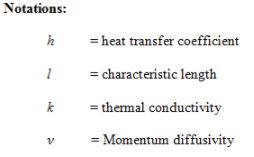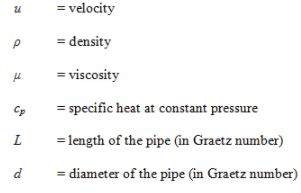4.3 Flow through a pipe or tube

4.3.1 Turbulent flow
A classical expression for calculating heat transfer in fully developed turbulent flow in smooth tubes/pipes of diameter (d) and length (L) is given by Dittus and Boelter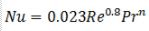(4.3)

where,

n = 0.4, for heating of the fluid

n = 0.3, for cooling of the fluid

The properties in this equation are evaluated at the average fluid bulk temperature. Therefore, the temperature difference between bulk fluid and the wall should not be significantly high.

Application of eq. 4.3 lies in the following limits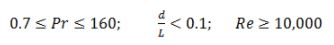Gnielinski suggested that better results for turbulent flow in smooth pipe may be obtained from the following relations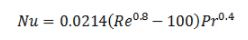(4.4)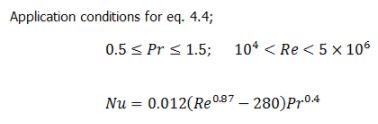(4.5)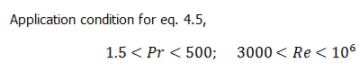When the temperature difference between bulk fluid and wall is very high, the viscosity of the fluid and thus the fluid properties changes substantially. Therefore, the viscosity correction must be accounted using Sieder – Tate equation given below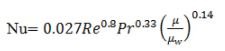(4.6)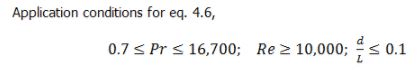However, the fluid properties have to be evaluated at the mean bulk temperature of the fluid except μw which should be evaluated at the wall temperature.
The earlier relations were applicable for fully developed flow when entrance length was negligible. Nusselt recommended the following relation for the entrance region when the flow is not fully developed.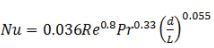(4.7)

where, L is the tube length and d is the tube diameter.

The fluid properties in eq. 4.7 should be evaluated at mean bulk temperature of the fluid.

Applicability conditions,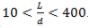.
As different temperature terms will appear in the course therefore to understand these terms see the following details.

Bulk temperature/mixing cup temperature: Average temperature in a cross-section.

Average bulk temperature: Arithmetic average temperature of inlet and outlet bulk temperatures.

Wall temperature: Temperature of the wall.

Film temperature: Arithmetic average temperature of the wall and free stream temperature.

Free stream temperature: Temperature free from the effect of wall.

Log mean temperature difference: It will be discussed in due course of time

The document Forced Convective Heat Transfer - 2 Notes | Study Heat Transfer - Mechanical Engineering is a part of the Mechanical Engineering Course Heat Transfer.
All you need of Mechanical Engineering at this link: Mechanical EngineeringUse Code STAYHOME200 and get INR 200 additional OFF

## Heat Transfer

58 videos|70 docs|85 tests

Track your progress, build streaks, highlight & save important lessons and more!

,

,

,

,

,

,

,

,

,

,

,

,

,

,

,

,

,

,

,

,

,

;